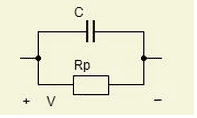# Parasitic effect of Capacitor question

Learnphysics

## Homework StatementA 68 nF capacitor has a parasitic parallel resistance Rp = 1 MΩ.
If a voltage V = Vmsin(ωt) is applied, as shown in the diagram, find the frequencies at which:
1. The current amplitude through the parasitic resistance is 1% of the current amplitude
through the capacitor.
2. The amplitudes of the currents through the resistor and the capacitor are equal.

## Homework Equations

I=C(dv/dt) Through capacitor

I=V/R Through Resistance.

## The Attempt at a Solution

I want to try to solve the second part of the question first because it would provide be a more fundamental solution.

In any case, I've equated the current through the capacitor with the current through the parasitic resistor:

Cdv/dt = V/Rp

Simple enough.

Now V=Vmsin(wt)
so
dv/dt = (w)(Vm)(Cos)(wt)

Substituting in we get:

(C)(w)(Vm)(Cos)(wt) = Vmsin(wt)/(Rp).

so:
(Rp)(C)(w)(Vm)(Cos)(wt) = Vmsin(wt) <-- just moving the resistance to the other side

At t=0. We know that sin waves have 0 amplitude, and cos waves have 1 amplitude. So setting t=0.

(Rp)(C)(w) = 0

Or in another words w=0, and frequency also =0.

Clearly that isn't correct though. What am I missing.

---------------------------------------
At the stage
Cdv/dt = V/Rp

I've tried integrating both sides with respect to dv and dt. which produces:

(C)(Rp)V = -(1/W)(V)(Cos(wt))
When t=0.

-(C)(Rp)(W) = 1
And we can solve for W
and thus frequency.

that produces the right answer, but I can't see why the first approach produces the answer f=0. I think the problem is to do with how I've used/understood calculus in the first attempt. Can anyone shed any light?

Homework Helper

## Homework StatementA 68 nF capacitor has a parasitic parallel resistance Rp = 1 MΩ.
If a voltage V = Vmsin(ωt) is applied, as shown in the diagram, find the frequencies at which:
1. The current amplitude through the parasitic resistance is 1% of the current amplitude
through the capacitor.
2. The amplitudes of the currents through the resistor and the capacitor are equal.

## Homework Equations

I=C(dv/dt) Through capacitor

I=V/R Through Resistance.

## The Attempt at a Solution

I want to try to solve the second part of the question first because it would provide be a more fundamental solution.

In any case, I've equated the current through the capacitor with the current through the parasitic resistor:

Cdv/dt = V/Rp

Simple enough.

Now V=Vmsin(wt)
so
dv/dt = (w)(Vm)(Cos)(wt)

Substituting in we get:

(C)(w)(Vm)(Cos)(wt) = Vmsin(wt)/(Rp).

so:
(Rp)(C)(w)(Vm)(Cos)(wt) = Vmsin(wt) <-- just moving the resistance to the other side

At t=0. We know that sin waves have 0 amplitude, and cos waves have 1 amplitude. So setting t=0.

(Rp)(C)(w) = 0

Or in another words w=0, and frequency also =0.

Clearly that isn't correct though. What am I missing.

---------------------------------------
At the stage
Cdv/dt = V/Rp

I've tried integrating both sides with respect to dv and dt. which produces:

(C)(Rp)V = -(1/W)(V)(Cos(wt))
When t=0.

-(C)(Rp)(W) = 1
And we can solve for W
and thus frequency.

that produces the right answer, but I can't see why the first approach produces the answer f=0. I think the problem is to do with how I've used/understood calculus in the first attempt. Can anyone shed any light?

The problem is that the currents they are referring to are not instantaneous currents at a particular time t, they are average currents or RMS (root mean square) currents. The instantaneous current ratio has no particular value. You need to square the currents and then average over a cycle then take the square root. The average of cos^2(wt) is equal to the average of sin^2(wt).

Last edited:
Homework Helper
Gold Member
You wrote
Ic = CdV/dt
Ir = V/R
but V = V0sin(wt)
and dV/dt = wV0cos(wt)

So just use the amplitudes of Ic and Ir:
wCV0 = 0.01V0/R or wC = 0.01/R for Ic = 0.01Ir
and wCV0 = V0/R or wC = 1/R for Ic = Ir.
Solve for w in both cases.